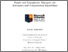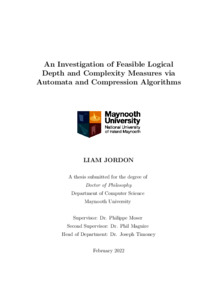# An Investigation of Feasible Logical Depth and Complexity Measures via Automata and Compression Algorithms

Jordon, Liam (2022) An Investigation of Feasible Logical Depth and Complexity Measures via Automata and Compression Algorithms. PhD thesis, National University of Ireland Maynooth.Previewmore...Add this article to your Mendeley library

## Abstract

When presented with a string or sequence of zeros and ones, that is an element of {0, 1}≤ω, it is often of interest to know how complex the object is. Was it created from some simple process or was it generated randomly? Kolmogorov Complexity is a fundamental tool of Algorithmic Information Theory which measures the complexity of such objects. However, there is one major problem: Kolmogorov Complexity is uncomputable. As such, the complexity of such objects are often studied in lower computable settings. This thesis aims to extend the study of such objects at lower complexity levels via finite-state automata, transducers and compression algorithms. We pay particular attention to normal sequences. One measurement which relies on Kolmogorov Complexity is Bennett’s Logical Depth, which has been described in the past as measuring how useful an object is. The primary objective of this thesis is to examine feasible notions of Bennett’s depth. We first extend an already studied infinitely often finite-state notion of depth to an almost everywhere notion. Secondly, we develop new notions based on pushdown compressors, the Lempel-Ziv 78 compression algorithm, and pebble transducers. We demonstrate the existence of deep sequences in each of these notions, and show which properties of Bennett’s original notion they satisfy. We also determine the differences between some of the depth notions we examine as follows: For a pair of depth notions (A, B), we construct a sequence S such that its A-depth level and B-depth level are unequal, i.e. S is ‘more’ deep in one notion than the other. This demonstrates that there is not a single unifying notion of depth. Our secondary objective is to examine normal sequences at lower complexity levels. Normal sequences are of interest to us as they are often considered finite- state random as they cannot be compressed by any information lossless finite-state compressor. We do this by, when appropriate, identifying normal sequences which are deep in the depth notions we develop. Furthermore, we prove the existence of a normal sequence which can be compressed by the PPM* compression algorithm which is incompressible by the Lempel-Ziv 78 algorithm. This, to our knowledge, is the first example of a mathematical proof differentiating the two algorithms on specific inputs as opposed to experimental results. We conclude by giving examples of normal sequences which have non-maximal automatic complexity, a complexity measurement based on finite-state automata.

Item Type: Thesis (PhD) Investigation; Feasible Logical Depth; Complexity Measures; Automata and Compression Algorithms; Faculty of Science and Engineering > Computer Science 16566 IR eTheses 22 Sep 2022 14:11

### Repository Staff Only(login required)Item control page